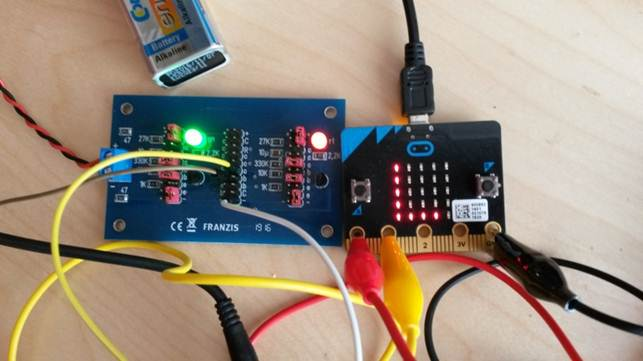#Dual transistor tester with the BBC micro:bit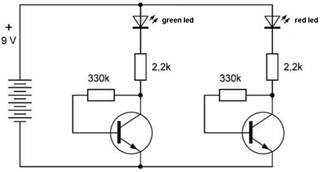The two transistors on the Lernpakket board are configured in collector feedback biasing.

By measuring the Vc's of each transistor, their gains -- being the ratios of the collector and bias currents ic and ib respectively, and assuming constant voltages with values Vbe = 0.6 V, Vgreenled = 2,45 V and Vredled = 1,85 V -- can be calculated.

In formulas:       gain = ic / ib
ib = (Vc - Vbe) / Rb
ic = (Vcc - Vled - Vc) / Rc

#The circuit

The mico:bit software

#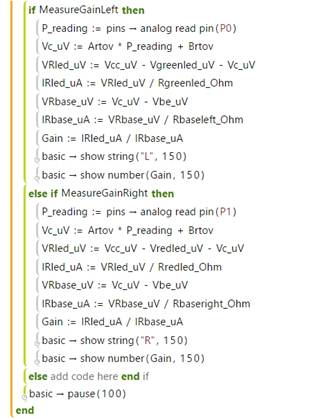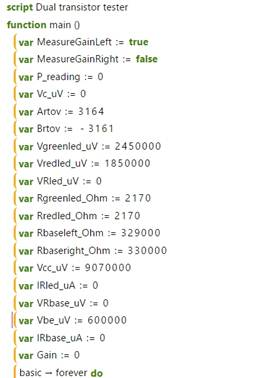Calibration of the ADC of the micro:bit

The micro:bit's ADC was calibrated using the stable voltage of a Li-cell and a zero voltage, the Li-cell supplying 2.93 V.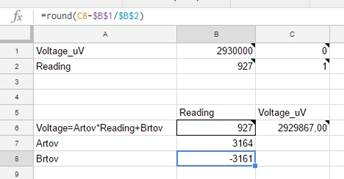Since the micro:bit deals with intergers only, voltages were expressed in microvolts, currents in microamps and resistances in ohms.

A linear relationship was used: Voltage in microvolts = 3164 * ADC_reading - 3161.

Results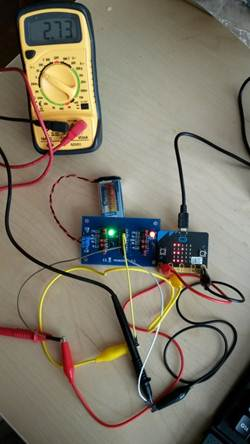On pressing the left button A of the micro:bit, the gain of the left transistor on the Franzis board is measured and displayed.
On pressing the right button B of the micro:bit, the gain of the right transistor on the Franzis board is measured and displayed.
The multimeter measures Vc of the left transistor, which is also measured on pin0 of the micro:bit.
The result of the gain measurement of the left transitor with the green led on the board under investigation was 298, that of the right transistor with the red led 281.